﻿ Dramatica: Inside the Clockwork
 For Story StructureFor Story Development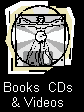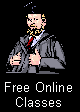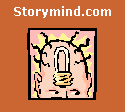Novel Writing SoftwareThousands of writers use StoryWeaver to build their story’s world, characters, plot, theme,
and genre.

Thousands of writers use Dramatica to find and refine their story’s structure and to find and fix holes and missteps.• 200 Interactive Story Cards guide you from concept to completion, step by step.
• Help Buttons with Writing Tips, Example Stories, Hints, and Tricks.
• Work on multiple stories at once.
• Jot down creative notes from anywhere in StoryWeaver.
• Placeholder: Pick up writing where you left off.
• Develop multiple levels of detail for your plot and characters.
• Works on any device: desktop, laptop, tablet, phone, Windows, Macintosh, iOs, Android & Chrome• Patented Story Engine cross- references your dramatic choices to create a structure map.
• AI style narrative model finds holes, missteps and suggests how to fix & fill them.
• Automatically generates a timeline from your story’s structural map.
• Offers three levels of detail depending on the complexity of your story.
• Includes hundreds of examples, tips, tricks, and techniques.
• Comes with more than sixty structural maps for classic and popular novels, movies and stage plays.Dramatica: Inside the Clockwork

By Melanie Anne Phillips
Creator StoryWeaver, Co-creator DramaticaIntroduction

While much has been written about the Dramatica Theory and Model of Story Structure, the focus has been primarily on how it works and how to apply it.  Yet every once and a while, someone asks what is to me a much more interesting question: Why does it work?  And even more rarely, someone of a more philosophical bent will ask and even more penetrating question: What does it mean?

In answer to these last two queries, I’ve gathered together the very best essays and articles I’ve written over the last quarter century about the inner workings of Dramatica – they whys and wherefores of its structure and function.

This book delves deeply into the reasoning behind the design and construction of the model, the underpinnings of theory beneath the level of narrative, and provides a view of the elegance an grandeur of Dramatica from a perspective seldom seen: from within the model itself.

We begin our journey by entering the model through its principal gateway, the quad, which leads us deeper and deeper into secret passages and wormholes as we explore a new point of view from which to appreciate Dramatica: inside the clockwork.

~ One ~

The Dramatica Structural Model

Here's an article I wrote about fifteen years ago that described the reason for and functioning of the Dramatica Table of Story Elements.  Though our understandings have refined over the years, the underlying concepts remain unchanged.

The Model of Dramatica

Just as the physical Periodic Table of the Elements is divided into families, such as the noble gasses or rare earths, so too is the Dramatica Table of Story Elements. Families occur in groups of four called Quads.

A Quad is much more than just a framework to hold story points. In fact, there are a number of relationships expressed by the quad form. By itself, the bare quad represents a linear equation. Each of the four smaller squares in the Quad represents one of the variables in the equation.

In addition, if one moves through all four variables in a Quad in a particular order, it adds a non-linear aspect to the Quad's list of functions. Also, by comparing and/or interacting the variables in the Quad, a relativity is described in which an overall equilibrium must be maintained keep the value of the Quad as a whole within prescribed limits.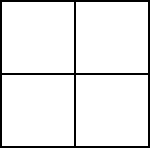So, a Quad functions in four ways simultaneously:

1. As a physical expression of linear equations.

2. As a temporal expression of non-linear equations.

3. As a conceptual expression of relativistic relationships.

4. As a framework for Appreciations, much like a Periodic Table of Elements.

To illustrate these four functions, we can assign an arbitrary name to each of the four variables so that we may write the forms of their relationships.

The Linear Form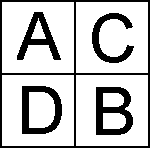A/B = CD

There are two ways to read this equation: as an objective description of the processes of the mind and alternatively as a subjective description of the experience of engaging in those processes.

The Objective interpretation of the equation reads (in conversational terms): When "A" is considered against "B", their relative value is measured against the product of "C" and "D" combined. Simply stated, this means that logic and emotion are co-dependent, for reasons we shall see later.

The same equation interpreted Subjectively reads: When "A" is held separate from "B", "C" and "D" will be blended. Simply stated, this means that the mind must blur the distinction between some items in order to define others.

Clearly, both interpretations are similar, but each casts the meaning of the equation in a slightly different light. Just as clearly, we are not using mathematical symbols in the same way one might in standard Algebra. In fact, the verbal description of the equation sounds more like a chemical function than a mathematical equation. As we shall soon explore, the Mental Relativity model of self-awareness describes the binary nature of neural firing as it relates to the biochemical impact of the environment surrounding neurons. Therefore, the mathematical model requires a symbolic means of expression that can accommodate both.

The Non-Linear Form

Rather than looking at a quality pertaining to a single Quad as we did with the linear form, we will be examining the permutation of a single Quad through several iterations which describe the non-linear form.

For reference, examine the following figure that illustrates the fully developed model as a whole once the Quad has gone through all of its iterations, which according to theory, ultimately bring it back to its initial values.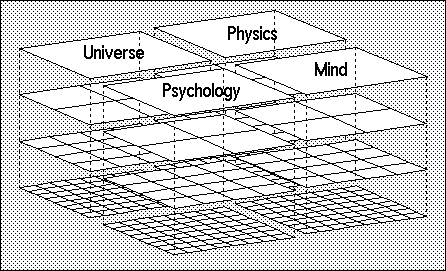The above model is made up entirely of Quads in a multi-dimensional matrix. The matrix is constructed by representing the spatial, fractal nature of Quads in the vertical plane, and by representing the temporal, frictal (dynamic fractal) nature of Quads in the horizontal plane. It is the interference pattern created by the intersection of the fractal and frictal relationships which expands to fill three-dimensional space in the model. Each position in the matrix, therefore, represents a different blend of fractal and frictal influences, and the matrix itself, therefore, forms the visual equivalent of a relativistic formula.

The non-linear nature of each Quad is seen in the iterations through the horizontal plane. For example, if we take our sample Quad of A,B,C, and D, we can move it through four iterations which effectively create a Quad of Quads, as illustrated below:Graphically, the first iteration is achieved by flipping the physical Quad like a page in a book from left to right. The resultant Quad still represents the form of the initial linear equation, A/B = CD, but the values of the variables have changed so that the equation now reads C/D = AB.

Iteration number 2 flips the new Quad over from top to bottom, arriving at a third set of values for the equation which now read B/A = DC.

Iteration number 3 flips the newest Quad from right to left creating a final, fourth set of values such that D/C = BA.

Iterations of Iterations

If we were to proceed through the final iteration (number 4) the Quad would return to its original position such that A/B = CD. At first it might appear that we have come full circle. But in fact, although each individual variable is back in its original place, their relationships have not returned to the original. This is because going "once around the track" with the position of the variables has actually changed the nature of the equation itself.

In fact, the equation has also gone through the first of four iterations such that it now reads:

A/C = DB

Whereas the initial equation compared diagonal relationships between variables (called Dynamic Pairs), the new equation compares horizontal relationships (Companion Pairs) between variables as illustrated below:

Initial Equation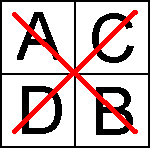Iterated EquationThe matrix of the model is constructed by representing this new equation as the first Quad in a new Quad of Quads, as illustrated below: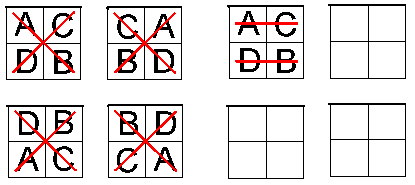Once again, the new Quad flips from left to right, top to bottom, and right to left, filling in the matrix until a second complete Quad of Quads is formed from equations which compare the horizontal variables.

When the equation in the new Quad of Quads finally returns to its initial orientation, it has once again been altered by the process. Now, although the variables still remain in their initial positions, the equation now compare them vertically, as shown below in Dependent Pairs: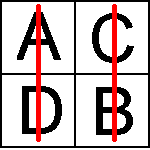Through its four iterations, this new equations adds another Quad of Quads in the "B" position of the horizontal plane. When it returns to its original orientation of variables, the equation has changed one final time. So far, the following three iterations have been explored:What pattern remains for the final Quad of Quads? Actually, there are two patterns remaining:The equation on the left serves to examine the four variables as individual elements, ignoring (for the moment) their similar qualities as a family. In this way, the unique qualities of each may be explored. Conversely, the equation represented on the right looks at nothing but the family characteristics, ignoring individual deviations entirely.

Just by looking at the relationships expressed by these two Quads, we can see a tangential difference in the internal dynamics compared to the pairs of the first three styles. In fact, this indicates a completely different set of functions performed by these Quads than we have previously seen.

For example, in the Quad on the left, we have already compared the value of the variable "A" to "B", "A" to "C", and "A" to "D", in the original three Quad forms by virtue of the pair relationships created in A/B = CD, and all of it's permutations. As earlier mentioned, this was an "Objective" reading of the equation.

Also, as we have already seen, in a "Subjective" interpretation, "A" and "B" are held separate, while "C" and "D" are blended. For example, "A" is compared to "CD", to "CB", and to "BC", and "B" is compared to "CD", to "AC", and to "AD".

In each case, the notion of an Objective and a Subjective view is accepted as a given, and rather than being built into the Quad is held as a responsibility of the Observer. Having fully explored the Quad relationships with this given, it is time to turn the tables on the Observer and compare the Objective to the Subjective. This is why the Quad dynamics appear quite unlike what has come before.

Let us examine the meaning held by each of these new Quad forms. We'll begin with the Independent Quad: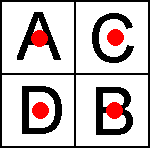The first iteration of this perspective is to see how "A" relates to the combined influence of "B", "C", and "D". There are two ways to calculate this influence. One way puts "A" outside of the product of "BCD" as illustrated below: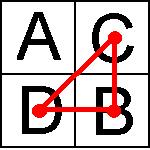This is a pseudo-objective relationship, illustrating that the observer at "A", seeks to approach Objectivity by excluding self from the equation. Clearly, from the previous Quads, we can see that "A" influences the other three variables in many ways. Therefore, rather than being a truly Objective view in which the Observer can actually stand apart from that which is observed, the Observer is always impacting the observation.

To carry it a step farther, as we read this page, we assume we are taking an Objective view of the Quad Dynamics without affecting them. If we accept the point of view of the reader as true Objectivity, then the view from "A" is pseudo-objectivity, and more like a Subjective view of the Objective.

In contrast, the Subjective component within the Quad itself appears as follows: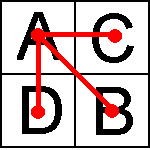Here, the Observer combines the influences of "AB", "AC", and "AD", and as a result, "A" is given equal weight with the product of "BCD". This is a pseudo-subjective view, for the truly Subjective view does not look outward at all. In a sense, the Observer at "A" examines how everything relates to him or her, but is not actually examining his or her self. So, rather than being truly Subjective, this approach is more like an Objective view of the Subjective.

The three-pronged pseudo-subjective pattern is referred to as a Splay, and the closed pseudo-objective pattern is called a Display.

Each Quad in the fourth and final Quad of Quads in the overall model represents both views. By the time it has gone through all of its permutations, each of the independent variables has been compared to all combinations of the other three, both Objectively and Subjectively, and the final Quad has been completed.

Still remaining is the Collective view, illustrated below: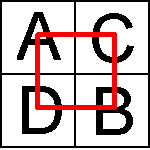This view has only one interpretation: we are no longer seeing the variables as individual units, but instead see only the result of the entire equation, all internal relationships taken into account. This Collective view essentially defines a family in which the Quad belongs. It is both the sum of the parts and an umbrella which covers all considerations falling under its heading.

Since we have already explored four Quads of Quads for a total of sixteen, we can project a pattern of sixteen family names, each of which describes and represents one Quad. In the model, these family names form a second level in the three-dimensional matrix, as illustrated below: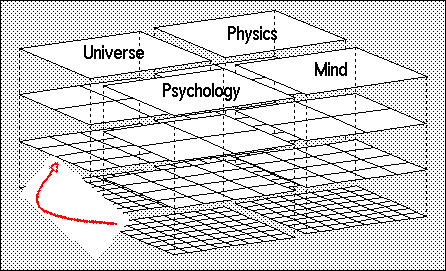The sixteen family names at the second level are called Variations. Each Quad of four Variations functions just like the Quads of Elements at the first level. Also each Quad of Variations is described by single family at an even higher level made up of family names called Types, represented in the third level up from the bottom of the model. Finally, each Quad of four Types culminates in a single family name called a Class.

It should be noted that by the time we have moved up four levels, the Collective approach to the Quad has been explored to the same degree as the diagonal (Dynamic) , horizontal (Companion), vertical (Dependent), and Independent comparisons, balancing them in an initial equilibrium.

This can be seen in the "weight" carried by the Collective iteration, not by how many elements it contains. To illustrate this, note the fact that although there are sixty-four Elements, there are only sixteen Variations, and four Types in a single Class. Here's why: Each Variation, being a collective, has the same "weight" as four Elements, because in fact it is composed or comprised of four Elements. So, sixteen Variations weigh as much as sixty-four Elements. Similarly, four Types also weigh as much as sixty-four Elements, which is the same weight as sixteen Variations. And finally, a single Class also has the weight of sixty-four Elements, or sixteen Variations, or four Types.

From this explanation, we can see that each level can be taken separately as another temporal iteration originating with a single quad at the Element level and carrying it to it's ultimate extent. In fact, it is a closed system from this perspective, though as we shall see later, from other perspectives it will appear to be an open system, best described as a quad-helix.

To recap then, we have iterated a single quad into a horizontal arrangement of sixty-four elements, then jumped into the vertical dimension and worked upwards from a wide particulate view to a singularity. In doing so, each iteration has not only carried the process along, but has also built up a "weight" which really represents "pre-processing" of future iterations. In other words, part of the substance of future iterations along the linear progression is accomplished in advance as the product of earlier iterations.

That this should exist is essential to the model. It represents a relativity among the operations so that no event which takes place in the iterative progression does so in a vacuum. Rather, it has a more holistic impact, not unlike the effect of gravity or the effect of the biochemistry of the brain as a medium across which the impact of a neuron firing is eventually felt by another neuron even though they have no direct synaptic connection.

In the model, the iterations from quad to quad of quads to set of sixty-four elements creates a progressively stronger impact on the next "step" to come. Therefore, with each iteration, there is less "control" available to that next iteration because the values of its variables and the natures of its operations have already been "weighted" to fall within certain limits.

Now, this would then give more weight to the earlier iterations than to the later ones, except for another essential component of the model, and that is bi-directionality. Rather than beginning at the bottom and working up, we could begin at the top and work down to create the structure.

In this approach, we start with a single item from a quad, such as the variable "A", which we have used in our generic representation of A, B, C, and D. If, rather than combining discrete particles into larger units we "deconstruct" an Elemental particle into smaller units, we move in an opposite direction.

The single Class item at the top of the iterative tower is exactly the same as an Element in any one of the quads in the structure, including the quad which began the original iteration at the bottom. The only difference among the quads is the arrangement of Elements within the quad, and the arrangement or contextual position of a given quad in the overall matrix (representing it's relativistic qualities in the structure as a whole).

So, beginning with a single item at the Class level, we can break it down into four component pieces represented by a quad containing a full complement of A,B,C, and D. From this, we can deconstruct each of the four components of the Type quad into four Variations each, also representing A,B,C, and D. Finally, each Variation can be broken down into four Elements, A,B,C, and D.

What we did originally when working up was to take a temporal or progressive view of the structure and follow the iterations to a point of singularity. What we have now done is to start with a singularity and take a spatial or component view of the structure, breaking each piece into smaller and smaller components as we work our way down to indivisible pieces.

Why are the Elements indivisible? Because when you start at a Class item and work your way down to the bottom level, one of the four Elements will be identical in nature and quality to the Class item itself. So, for example, starting with a Class item with a quality of "A", we find at the bottom Element level a quad with an A,B,C, and D in which the Elemental "A" has the same nature as a starting point of iteration and the same contextual quality of position as the Class item A. In other words, the temporal and spatial qualities, or put another way, the progressive and relativistic qualities are identical between Class "A" and Element "A".

When looking at a single Class "tower", we can easily see each of the two "A"'s a being starting points. But, it does not at first appear that they share identical context. This becomes clear, however, when take into account that there are actually four Class towers (or matrices), each one beginning with a different Element at the top. So, there is an "A" Class, a "B" Class, a "C" Class, and a "D" Class, each at the top of a different matrix.

The four matrices are not independent, however, but represent collectively the full extent of "arguing" a single quad down to it's smallest components - smallest because at the bottom of each matrix, there will be a single quad which is identical in content and alignment to the initial quad made up of the four Class items at the top of the quad-matrix. That quad at the bottom of each of the four matrices is also the beginning point for each matrix in the iterative progression that works from the bottom up.

It all begins to look a little recursive, and from this perspective it is. Again, it only appears recursive when it is perceived as a closed system. In other articles, I describe the dynamics which rearrange the alignment of the items in the four matrices. These dynamics represent the process approach to the model, from which perspective it appears as an open-ended, ongoing iteration which never exactly returns to the point of origin.

Some final thoughts about the structural matrices before we conclude our Introduction to Quad Structure....

Because each of the four Class matrices starts at the top with a different Element as the "seed", one finds that the alignment of the Elements in each of the quads on the lower level reflects that initial starting point. In other words, one might look at the matrix creation process as being iterative, and if we seed the function with a value of "A", it will create a matrix that is identical in structure but different in arrangement of content than if we had begun with a value of "B", for example.

Still, all four Elements, A, B, C, and D must appear in every quad in all four matrices. So, the "filtering" effect of "looking" at the lower levels through the "perspective" of the Element which is the Class item at the top makes itself manifest not in different content (which must still be the four Elements) but in different arrangements of the Elements within the quads.

For example, the quads in the "B" matrix would place the "B" Element in the upper left hand corner of each quad, rather than the "A" Element as in the "A" matrix which we have illustrated in detail. But, just as when the progressive iteration from bottom to top shifts from the Element level to the Variation level and enters the vertical dimension, the nature of the realignment of Elements in the matrix changes when we move from top to bottom and arrive at the bottom Element level.

Note that when moving to the Variation level from the bottom, we indicated that most of the influences had already been pre-determined by the relativistic effects of the earlier iterations. And, in fact, we had already described the Dynamic, Companion, Dependent, and Independent aspects of Elemental relationships, leaving only the Collective relationship to be explored. So, the entire vertical structure, when seen from the bottom up approach pertains only to that last 1/2 of the fourth kind of pairing, the Collective.

Similarly, when looking from the top down, the last level at the bottom pertains only to the final aspect of deconstruction. Down to this point from the top, we have dealt with the arrangement of quads by noting their movement as Elements. But the "perspective shift" that occurs at the bottom Element level shifts for the first time into the binary. Rather than rearranging the quads as four Elements, the shifting occurs among bonded pairs comprised of two Elements each.

These bonded pairs do not rearrange at the Element level within a quad, but within a group of four quads. So, looking at the element levels of each of the four matrices, we see that the make-up of each bonded pair remains the same, but the position of the bonded pairs relative to those in the other matrices is different.

The repositioning of bonded pairs within the quads of quads is the result of the "filtering" effect caused by the iterative deconstruction from top to bottom. Conversely, that each matrix is topped by a Class item that is a different Element, is the result of the shift caused by seeding the progressive iteration with a different arrangement of bonded pairs. Taken together they represent a unified model of the structure of the mind.

But the model is not yet complete. As it stands, the model is fixed and inanimate; hardly in line with the dynamic nature of the mind. In fact, structure is only half of the Mental Relativity model. The other half are the algorithms which describe how the components of the structure are rearranged, much as one might twist up a Rubik's cube, how this is driven by external and internal stimuli, and how it builds up potential, much like winding a clock.

While the brain may be described in terms of its components, the mind is a machine made of time. Only when both the structural and dynamic aspects of the model are brought together can the mind/brain connection be drawn in such a way as to create a model which can both describe and predict.

In summary, we can see all around us reflections or harmonics of some of these concepts. That we see the external world as being most essentially described at a macroscopic level as being comprised of Mass, Energy, Space, and Time, that we see at a microscopic level the building blocks of existing as being Gravity, Electromagnetism, Strong, and Weak forces, that we see four bases in DNA and that they combine in bonded pairs, that we see a spiral in a Galaxy and in a Teacup, should not surprise us. For in the end, the order we see in the universe is projected by our own minds, and if we look as deeply as we can at anything, we will ultimately see no more than ourselves staring back. To look most deeply into the universe it to look into a mirror.

At this point, we have fulfilled the purpose of this article, which was to provide an introduction to Quad structure. Other articles describe the mechanism by which the model is dynamically rearranged, how that reflects the functioning of our mental processes, the biologic basis of self-awareness, and the practical application of the Mental Relativity theory and model to everyday concerns.

*****

We hope you have enjoyed this sample chapter of the 202 page book.

Purchase the entire book in Paperback \$14.95 or Kindle \$5.99

Dramatica: Inside the Clockwork